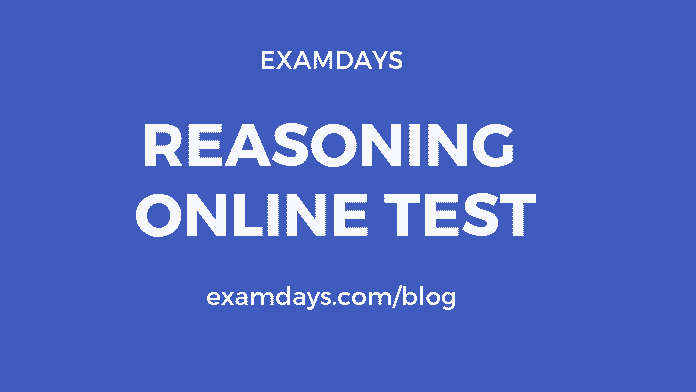# Reasoning Online Test Quiz #6 with Answers

0# Reasoning Online Test

Logical Reasoning Online Test With Answers: The Logical reasoning questions are given for the practice purpose, this reasoning online test is useful for the various examinations like RRB, SSC, Banks(IBPS), PSU, and other important State-wise examinations

Q1. Find the next number in the sequence: 30, 45, 90, 225, 675

1. 1890
2. 1745
3. 2362.5
4. 1845
5. 1564

2362.5

Q2.  Find the next number in the sequence: 190, 94, 46, 22, 10, 4

1. 0.4
2. 0.6
3. 1.5
4. 1.6
5. 1.0

1.0

Q3. Mayank walks faster than Gurjot.
Lalit walks faster than Mayank.
Gurjot walks faster than Lalit.
If the first two statements are true, the third statement is

1. True
2. False
3. Maybe
4. None of these

TRUE

Q4. Tanuj is older than Eina.
Chetan is older than Tanuj.
Eina is older than Chetan.
If the first 2 statements are true, the 3rd statement is

1. True
2. False
3. Maybe
4. None of these

False

Q5. A man purchased 15 pens, 12 books, 10 pencils and 5 erasers. The cost of each pen is Rs.36, each book is Rs.45, each pencil is Rs.8, and the cost of each eraser is Rs.40 less than the combined costs of pen and pencil. Find the total amount spent?

1. 1600
2. 1520
3. 1560
4. 1540
5. 1522

1560

Q6. Find a pair that is similar to the given pair of numbers:- 180:90

• 64 : 37
• 2:1
• 23 : 10
• 137 : 112

2:1

Q7. If in each number first digit is replaced by the second digit, the second digit is replaced by the third digit and third digit is replaced by the first digit, then which number will be the second-lowest?

1. 625
2. 843
3. 236
4. 758
5. 417

236

Q8. 625 843 236 758 417; If in each number, the first and the third digits are interchanged, and then which number will be the highest?

1. 843
2. 417
3. 236
4. 758
5. 645

417

Q9. If each consonant in the word “ELEPHANT” is changed to the previous letter in the English alphabetical series and each vowel is changed to the next letter in the English alphabetical series, and then the alphabets so formed are arranged in an alphabetical order from left to right, which will be the third from the right?

1. F
2. O
3. S
4. G
5. M

S

Q10.  How many such pairs of letters are there in the word MODERN, each of which has as many letters between them in the word (in both forward and backward directions) as they have between them in the English alphabetical series?

1. None
2. 2
3. 4
4. 5
5. 6